BAYES Statement
BAYES <options> ;
The BAYES statement requests a Bayesian analysis of the regression model by using Gibbs sampling. The Bayesian posterior samples (also known as the chain) for the regression parameters can be output to a SAS data set. Table 66.2 summarizes the options available in the BAYES statement.
Table 66.2 BAYES Statement Options

Option

Description

Monte Carlo Options

Specifies initial values of the chain

Specifies the number of burn-in iterations

Specifies the number of iterations after burn-in

Specifies the sampling algorithm

Specifies the random number generator seed

Sontrols the thinning of the Markov chain

Model and Prior Options

Specifies the prior of the regression coefficients

Specifies details of the piecewise exponential model

Summaries and Diagnostics of the Posterior Samples

Displays convergence diagnostics

Displays diagnostic plots

Displays summary statistics

Posterior Samples

Names a SAS data set for the posterior samples

The following list describes these options and their suboptions.

COEFFPRIOR=UNIFORM | NORMAL <(normal-option)> |  ZELLNER <(zellner-option)>
CPRIOR=UNIFORM | NORMAL <(normal-option)>  | ZELLNER <(zellner-option)>
COEFF=UNIFORM | NORMAL <(normal-option)> | ZELLNER <(zellner-option)>
specifies the prior distribution for the regression coefficients. The default is COEFFPRIOR=UNIFORM. The following prior distributions are available:
UNIFORM

specifies a flat prior—that is, the prior that is proportional to a constant (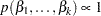for all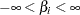).

NORMAL<(normal-option)>

specifies a normal distribution. The normal-options include the following:

INPUT=SAS-data-set

specifies a SAS data set that contains the mean and covariance information of the normal prior. The data set must contain the _TYPE_ variable to identify the observation type, and it must contain a variable to represent each regression coefficient. If the data set also contains the _NAME_ variable, values of this variable are used to identify the covariances for the _TYPE_=’COV’ observations; otherwise, the _TYPE_=’COV’ observations are assumed to be in the same order as the explanatory variables in the MODEL statement. PROC PHREG reads the mean vector from the observation with _TYPE_=’MEAN’ and the covariance matrix from observations with _TYPE_=’COV’. For an independent normal prior, the variances can be specified with _TYPE_=’VAR’; alternatively, the precisions (inverse of the variances) can be specified with _TYPE_=’PRECISION’.

RELVAR <=c>

specifies a normal prior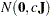, where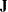is a diagonal matrix with diagonal elements equal to the variances of the corresponding ML estimator. By default, c=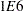.

VAR=c

specifies the normal prior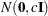, whereis the identity matrix.

If you do not specify a normal-option, the normal prior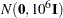, whereis the identity matrix, is used. See the section Normal Prior for details.
ZELLNER<(zellner-option)>
specifies the Zellner g-prior for the regression coefficients. The g-prior is a normal prior distribution with mean zero and covariance matrix equal to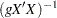, where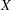is the design matrix andcan be a constant or a parameter with a gamma prior. The zellner-options include the following:
G=number

specifies a constant number for.

GAMMA <(SHAPE=a ISCALE=b)>

specifies thathas a gamma prior distribution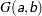with density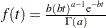. By default, a=b=1E–4.

If you do not specify a zellner-option, the default is ZELLNER(g=1E–6).
DIAGNOSTICS=ALL | NONE | keyword | (keyword-list)
DIAG=ALL | NONE | keyword | (keyword-list)
controls the number of diagnostics produced. You can request all the diagnostics in the following list by specifying DIAGNOSTICS=ALL. If you do not want any of these diagnostics, you specify DIAGNOSTICS=NONE. If you want some but not all of the diagnostics, or if you want to change certain settings of these diagnostics, you specify a subset of the following keywords. The default is DIAGNOSTICS=(AUTOCORR GEWEKE ESS).
AUTOCORR <(LAGS= numeric-list)>

computes the autocorrelations of lags given by LAGS= list for each parameter. Elements in the list are truncated to integers and repeated values are removed. If the LAGS= option is not specified, autocorrections of lags 1, 5, 10, and 50 are computed for each variable. See the section Autocorrelations for details.

ESS

computes the effective sample size of Kass et al. (1998), the correlation time, and the efficiency of the chain for each parameter. See the section Effective Sample Size for details.

MCSE
MCERROR

computes the Monte Carlo standard error for each parameter. The Monte Caro standard error, which measures the simulation accuracy, is the standard error of the posterior mean estimate and is calculated as the posterior standard deviation divided by the square root of the effective sample size. See the section Standard Error of the Mean Estimate for details.

HEIDELBERGER <(heidel-options)>
computes the Heidelberger and Welch tests for each parameter. See the section Heidelberger and Welch Diagnostics for details. The tests consist of a stationary test and a halfwidth test. The former tests the null hypothesis that the sample values form a stationary process. If the stationarity test is passed, a halfwidth test is then carried out. Optionally, you can specify one or more of the following heidel-options:
SALPHA=value

specifies thelevel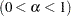for the stationarity test. The default is the value of the ALPHA= option in the PROC PHREG statement, or 0.05 if that option is not specified.

HALPHA=value

specifies thelevelfor the halfwidth test. The default is the value of the ALPHA= option in the PROC PHREG statement, or 0.05 if that option is not specified.

EPS=value

specifies a small positive numbersuch that if the halfwidth is less thantimes the sample mean of the retaining samples, the halfwidth test is passed.

GELMAN <(gelman-options)>
computes the Gelman and Rubin convergence diagnostics. See the section Gelman and Rubin Diagnostics for details. You can specify one or more of the following gelman-options:
NCHAIN=number
N=number

specifies the number of parallel chains used to compute the diagnostic and has to be 2 or larger. The default is NCHAIN=3. The NCHAIN= option is ignored when the INITIAL= option is specified in the BAYES statement, and in such a case, the number of parallel chains is determined by the number of valid observations in the INITIAL= data set.

ALPHA=value

specifies the significance level for the upper bound. The default is the value of the ALPHA= option in the PROC PHREG statement, or 0.05 if that option is not specified (resulting in a 97.5% bound).

GEWEKE <geweke-options>
computes the Geweke diagnostics. See the section Geweke Diagnostics for details. The diagnostic is essentially a two-sample-test between the first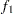portion and the last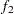portion of the chain. The default is=0.1 and=0.5, but you can choose other fractions by using the following geweke-options:
FRAC1=value

specifies the earlyfraction of the Markov chain.

FRAC2=value

specifies the latterfraction of the Markov chain.

RAFTERY <(raftery-options)>
computes the Raftery and Lewis diagnostics. See the section Raftery and Lewis Diagnostics for details. The diagnostic evaluates the accuracy of the estimated quantile (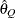for a given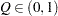) of a chain.can achieve any degree of accuracy when the chain is allowed to run for a long time. A stopping criterion is when the estimated probability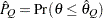reaches within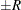of the value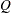with probability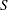; that is,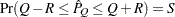. The following raftery-options enable you to specify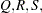and a precision levelfor a stationary test.
QUANTILE=value
Q=value

specifies the order (a value between 0 and 1) of the quantile of interest. The default is 0.025.

ACCURACY=value
R=value

specifies a small positive number as the margin of error for measuring the accuracy of estimation of the quantile. The default is 0.005.

PROBABILITY=value
P=value

specifies the probability of attaining the accuracy of the estimation of the quantile. The default is 0.95.

EPSILON=value
EPS=value

specifies the tolerance level (a small positive number) for the test. The default is 0.001.

INITIAL=SAS-data-set

specifies the SAS data set that contains the initial values of the Markov chains. The INITIAL= data set must contain a variable for each parameter in the model. You can specify multiple rows as the initial values of the parallel chains for the Gelman-Rubin statistics, but posterior summary statistics, diagnostics, and plots are computed only for the first chain.

NBI=number

specifies the number of burn-in iterations before the chains are saved. The default is 2000.

NMC=number

specifies the number of iterations after the burn-in. The default is 10000.

OUTPOST=SAS-data-set
OUT=SAS-data-set

names the SAS data set that contains the posterior samples. See the section OUTPOST= Output Data Set in the BAYES Statement for more information. Alternatively, you can output the posterior samples into a data set, as shown in the following example in which the data set is named PostSamp.

```ODS OUTPUT PosteriorSample = PostSamp;
```
PIECEWISE <=keyword <(<NINTERVAL=number> <INTERVAL=(numeric-list)> <PRIOR=option>)>>
specifies that the piecewise constant baseline hazard model be used in the Bayesian analysis. You can specify one of the following two keywords:
HAZARD

models the baseline hazard parameters in the original scale. The hazard parameters are named Lambda1, Lambda2,, and so on.

LOGHAZARD

models the baseline hazard parameters in the log scale. The log-hazard parameters are named Alpha1, Alpha2,, and so on.

Specifying PIECEWISE by itself is the same as specifying PIECEWISE=LOGHAZARD.

You can choose one of the following two options to specify the partition of the time axis into intervals of constant baseline hazards:

NINTERVAL=number
N=number

specifies the number of intervals with constant baseline hazard rates. PROC PHREG partitions the time axis into the given number of intervals with approximately equal number of events in each interval.

INTERVAL=(numeric-list)

specifies the list of numbers that partition the time axis into disjoint intervals with constant baseline hazard in each interval. For example, INTERVAL=(100, 150, 200, 250, 300) specifies a model with a constant hazard in the intervals [0,100), [100,150), [150,200), [200,250), [250,300), and [300,). Each interval must contain at least one event; otherwise, the posterior distribution can be improper, and inferences cannot be derived from an improper posterior distribution.

If neither NINTERVAL= nor INTERVAL= is specified, the default is NINTERVAL=8.

To specify the prior for the baseline hazards (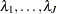) in the original scale, you specify the following:

PRIOR = IMPROPER | UNIFORM | GAMMA<(gamma-option)> |  ARGAMMA<(argamma-option)>

The default is PRIOR=IMPROPER. The available prior options include the following:

IMPROPER

specifies the noninformative and improper prior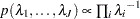for all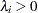.

UNIFORM

specifies a uniform prior on the real line; that is,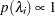for all.

GAMMA <(gamma-option)>

specifies an independent gamma prior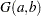with density, which can be followed by one of the following gamma-options enclosed in parentheses. The hyperparametersandare the shape and inverse-scale parameters of the gamma distribution, respectively. See the section Independent Gamma Prior for details. The default is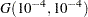for each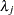, setting the prior mean to 1 with variance 1E4. This prior is proper and reasonably noninformative.

INPUT=SAS-data-set

specifies a data set containing the hyperparameters of the independent gamma prior. The data set must contain the _TYPE_ variable to identify the observation type, and it must contain the variables named Lambda1, Lambda2, ..., and so forth, to represent the hazard parameters. The observation with _TYPE_=’SHAPE’ identifies the shape parameters, and the observation with _TYPE_=’ISCALE’ identifies the inverse-scale parameters.

RELSHAPE<=c>

specifies independent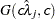distribution, where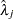’s are the MLEs of the hazard rates. This prior has meanand variance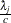. By default, c=1E–4.

SHAPE=a and ISCALE=b

together specify theprior.

SHAPE=c
ISCALE=c

specifies the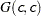prior.

ARGAMMA <(argamma-option)>
specifies an autoregressive gamma prior of order 1, which can be followed by one of the following argamma-options. See the section AR1 Prior for details.
INPUT=SAS-data-set

specifies a data set containing the hyperparameters of the correlated gamma prior. The data set must contain the _TYPE_ variable to identify the observation type, and it must contain the variables named Lambda1, Lambda2, ..., and so forth, to represent the hazard parameters. The observation with _TYPE_=’SHAPE’ identifies the shape parameters, and the observation with _TYPE_=’ISCALE’ identifies the relative inverse-scale parameters; that is, ifand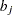are, respectively, the SHAPE and ISCALE values for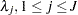, then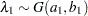, and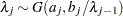for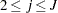.

SHAPE=a and SCALE=b

together specify that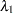and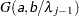for.

SHAPE=c
ISCALE=c

specifies that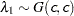and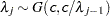for.

To specify the prior for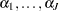, the hazard parameters in the log scale, you specifying the following:

PRIOR=UNIFORM | NORMAL<(normal-option)>
specifies the prior for the loghazard parameters. The default is PRIOR=UNIFORM. The available PRIOR= options are as follows:
UNIFORM

specifies the uniform prior on the real line; that is,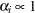for all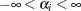.

NORMAL<(normal-option)>

specifies a normal prior distribution on the log-hazard parameters. The normal-options include the following. If you do not specify a normal-option, the normal prior, whereis the identity matrix, is used.

INPUT=SAS-data-set

specifies a SAS data set containing the mean and covariance information of the normal prior. The data set must contain the _TYPE_ variable to identify the observation type, and it must contain variables named Alpha1, Alpha2, ..., and so forth, to represent the log-hazard parameters. If the data set also contains the _NAME_ variable, the value of this variable will be used to identify the covariances for the _TYPE_=’COV’ observations; otherwise, the _TYPE_=’COV’ observations are assumed to be in the same order as the explanatory variables in the MODEL statement. PROC PHREG reads the mean vector from the observation with _TYPE_=’MEAN’ and the covariance matrix from observations with _TYPE_=’COV’. See the section Normal Prior for details. For an independent normal prior, the variances can be specified with _TYPE_=’VAR’; alternatively, the precisions (inverse of the variances) can be specified with _TYPE_=’PRECISION’.

If you have a joint normal prior for the log-hazard parameters and the regression coefficients, specify the same data set containing the mean and covariance information of the multivariate normal distribution in both the COEFFPRIOR=NORMAL(INPUT=) and the PIECEWISE=LOGHAZARD(PRIOR=NORMAL(INPUT=)) options. See the section Joint Multivariate Normal Prior for Log-Hazards and Regression Coefficients for details.

RELVAR <=c>

specifies the normal prior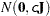, whereis a diagonal matrix with diagonal elements equal to the variances of the corresponding ML estimator. By default, c=1E6.

VAR=c

specifies the normal prior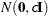, whereis the identity matrix.

PLOTS <(global-plot-options)> = plot-request
PLOTS <(global-plot-options)> = (plot-requests)

controls the diagnostic plots produced through ODS Graphics. Three types of plots can be requested: trace plots, autocorrelation function plots, and kernel density plots. By default, the plots are displayed in panels unless the global plot option UNPACK is specified. If you specify more than one type of plots, the plots are displayed by parameters unless the global plot option GROUPBY=TYPE is specified. When you specify only one plot request, you can omit the parentheses around the plot request. For example:

``` plots=none
plots(unpack)=trace
plots=(trace autocorr)
```

ODS Graphics must be enabled before requesting plots. For example:

``` ods graphics on;
proc phreg;
model y=x;
bayes plots=trace;
run;
end;
ods graphics off;
```

If ODS Graphics is enabled but you do not specify the PLOTS= option in the BAYES statement, then PROC PHREG produces, for each parameter, a panel that contains the trace plot, the autocorrelation function plot, and the density plot. This is equivalent to specifying plots=(trace autocorr density).

The global-plot-options include the following:

FRINGE

creates a fringe plot on the X axis of the density plot.

GROUPBY = PARAMETER | TYPE
specifies how the plots are to be grouped when there is more than one type of plots. The choices are as follows:
TYPE

specifies that the plots be grouped by type.

PARAMETER

specifies that the plots be grouped by parameter.

GROUPBY=PARAMETER is the default.
SMOOTH

displays a fitted penalized B-spline curve each trace plot.

UNPACKPANEL
UNPACK

specifies that all paneled plots be unpacked, meaning that each plot in a panel is displayed separately.

The plot-requests include the following:

ALL

specifies all types of plots. PLOTS=ALL is equivalent to specifying PLOTS=(TRACE AUTOCORR DENSITY).

AUTOCORR

displays the autocorrelation function plots for the parameters.

DENSITY

displays the kernel density plots for the parameters.

NONE

suppresses all diagnostic plots.

TRACE

displays the trace plots for the parameters. See the section Visual Analysis via Trace Plots for details.

Consider a model with four parameters, X1–X4. Displays for various specification are depicted as follows.

1. PLOTS=(TRACE AUTOCORR) displays the trace and autocorrelation plots for each parameter side by side with two parameters per panel:

 Display 1 Trace(X1) Autocorr(X1) Trace(X2) Autocorr(X2) Display 2 Trace(X3) Autocorr(X3) Trace(X4) Autocorr(X4)
2. PLOTS(GROUPBY=TYPE)=(TRACE AUTOCORR) displays all the paneled trace plots, followed by panels of autocorrelation plots:

 Display 1 Trace(X1) Trace(X2) Display 2 Trace(X3) Trace(X4) Display 3 Autocorr(X1) Autocorr(X2) Autocorr(X3) Autocorr(X4)
3. PLOTS(UNPACK)=(TRACE AUTOCORR) displays a separate trace plot and a separate correlation plot, parameter by parameter:

 Display 1 Trace(X1) Display 2 Autocorr(X1) Display 3 Trace(X2) Display 4 Autocorr(X2) Display 5 Trace(X3) Display 6 Autocorr(X3) Display 7 Trace(X4) Display 8 Autocorr(X4)
4. PLOTS(UNPACK GROUPBY=TYPE) = (TRACE AUTOCORR) displays all the separate trace plots followed by the separate autocorrelation plots:

 Display 1 Trace(X1) Display 2 Trace(X2) Display 3 Trace(X3) Display 4 Trace(X4) Display 5 Autocorr(X1) Display 6 Autocorr(X2) Display 7 Autocorr(X3) Display 8 Autocorr(X4)
SAMPLING=keyword
specifies the sampling algorithm used in the Markov chain Monte Carlo (MCMC) simulations. Two sampling algorithms are available:
ARMS
GIBBS

requests the use of the adaptive rejection Metropolis sampling (ARMS) algorithm to draw the Gibbs samples. ALGORITHM=ARMS is the default.

RWM

requests the use of the random walk Metropolis (RWM) algorithm to draw the samples.

For details about the MCMC sampling algorithms, see the section Markov Chain Monte Carlo Method in Chapter 7, Introduction to Bayesian Analysis Procedures.
SEED=number

specifies an integer seed ranging from 1 to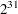–1 for the random number generator in the simulation. Specifying a seed enables you to reproduce identical Markov chains for the same specification. If the SEED= option is not specified, or if you specify a nonpositive seed, a random seed is derived from the time of day.

STATISTICS <(global-options)> = ALL | NONE | keyword | (keyword-list)
STATS <(global-statoptions)> = ALL | NONE | keyword | (keyword-list)
controls the number of posterior statistics produced. Specifying STATISTICS=ALL is equivalent to specifying STATISTICS=(SUMMARY INTERVAL COV CORR). If you do not want any posterior statistics, you specify STATISTICS=NONE. The default is STATISTICS=(SUMMARY INTERVAL). See the section Summary Statistics for details. The global-options include the following:
ALPHA=numeric-list

controls the probabilities of the credible intervals. The ALPHA= values must be between 0 and 1. Each ALPHA= value produces a pair of 100(1–ALPHA)% equal-tail and HPD intervals for each parameters. The default is the value of the ALPHA= option in the PROC PHREG statement, or 0.05 if that option is not specified (yielding the 95% credible intervals for each parameter).

PERCENT=numeric-list

requests the percentile points of the posterior samples. The PERCENT= values must be between 0 and 100. The default is PERCENT= 25, 50, 75, which yield the 25th, 50th, and 75th percentile points for each parameter.

You can specify the following values for a keyword or as part of a keyword-list. To specify a list, place parentheses around multiple keywords that are separated by spaces.
CORR

produces the posterior correlation matrix.

COV

produces the posterior covariance matrix.

SUMMARY

produces the means, standard deviations, and percentile points for the posterior samples. The default is to produce the 25th, 50th, and 75th percentile points, but you can use the global PERCENT= option to request specific percentile points.

INTERVAL

produces equal-tail credible intervals and HPD intervals. The defult is to produce the 95% equal-tail credible intervals and 95% HPD intervals, but you can use the global ALPHA= option to request intervals of any probabilities.

THINNING=number
THIN=number

controls the thinning of the Markov chain. Only one in everysamples is used when THINNING=, and if NBI=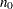and NMC=, the number of samples kept is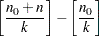where [] represents the integer part of the number. The default is THINNING=1.